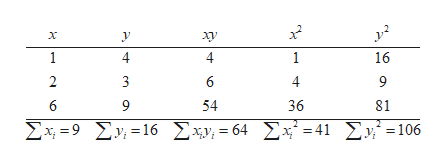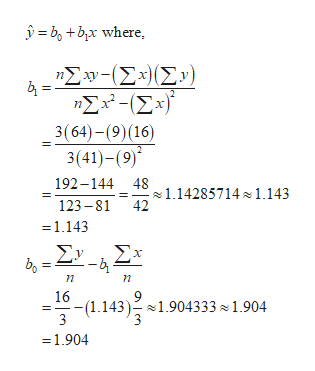Suppose you are given the following (x, y) data pairs.x126y439Find the least-squares equation for these data (rounded to three digits after the decimal).ŷ =  +  x(b) Now suppose you are given these (x, y) data pairs.x439y126Find the least-squares equation for these data (rounded to three digits after the decimal).ŷ =  +  x (c) In the data for parts (a) and (b), did we simply exchange the x and y values of each data pair?YesNo    (d) Solve your answer from part (a) for x (rounded to three digits after the decimal).x =  +  y Do you get the least-squares equation of part (b) with the symbols x and yexchanged?YesNo    (e) In general, suppose we have the least-squares equation y = a + bx for a set of data pairs (x, y). If we solve this equation for x, will we necessarily get the least-squares equation for the set of data pairs (y, x), (with x and y exchanged)? Explain using parts (a) through (d).In general, switching x and y values produces the same least-squares equation.Switching x and y values sometimes produces the same least-squares equation and sometimes it is different.    In general, switching x and y values produces a different least-squares equation.

Question

Suppose you are given the following (xy) data pairs.

 x 1 2 6 y 4 3 9

Find the least-squares equation for these data (rounded to three digits after the decimal).
ŷ =  +  x

(b) Now suppose you are given these (xy) data pairs.

 x 4 3 9 y 1 2 6

Find the least-squares equation for these data (rounded to three digits after the decimal).
ŷ =  +  x

(c) In the data for parts (a) and (b), did we simply exchange the x and y values of each data pair?

YesNo

(d) Solve your answer from part (a) for x (rounded to three digits after the decimal).
x =  +  y

Do you get the least-squares equation of part (b) with the symbols x and yexchanged?

YesNo

(e) In general, suppose we have the least-squares equation y = a + bx for a set of data pairs (xy). If we solve this equation for x, will we necessarily get the least-squares equation for the set of data pairs (yx), (with x and y exchanged)? Explain using parts (a) through (d).

In general, switching x and y values produces the same least-squares equation.Switching x and y values sometimes produces the same least-squares equation and sometimes it is different.    In general, switching x and y values produces a different least-squares equation.
Step 1

(a)

Consider the given data and calculate the following:help_outlineImage TranscriptioncloseΧ ν Χ ν 1 1 16 2 3 6 4 9 6 9 54 36 81 ΣΧ9Σ) =16Σy, 64 ΣΧ41 ΣΕ106 fullscreen
Step 2

Now, Least Squares Regression equation is given by:help_outlineImage TranscriptioncloseΣw-(Σ) (Σ) ηΣέ-Σ 3( 64)-(9) 16) 3(41)-(9) 192-144 48 1.14285714 1.143 123-81 42 =1.143 ΣΥ bg ΣΧ --b η η 16 -(1.1431.904333 1.904 3 3 1.904 fullscreen
Step 3

Now, put the values b0 and b1 and the Least Squares Regression...

Want to see the full answer?

See Solution

Want to see this answer and more?

Our solutions are written by experts, many with advanced degrees, and available 24/7

See Solution
Tagged in

Probability